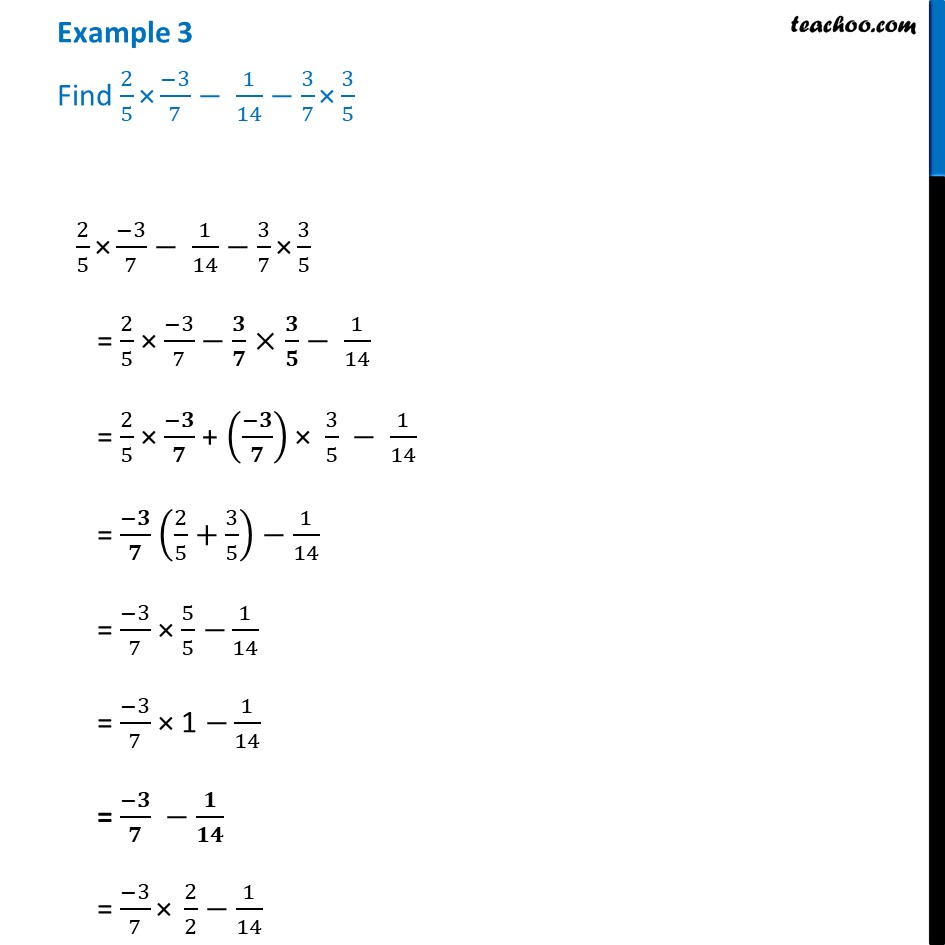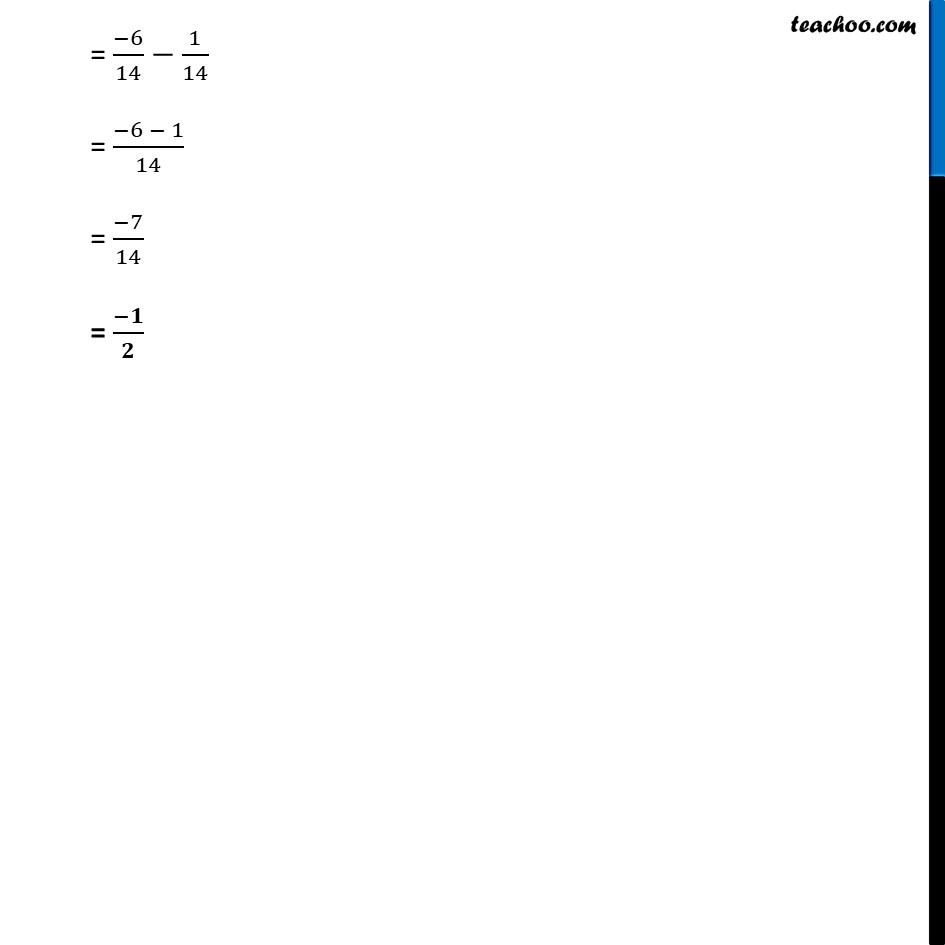Examples

Chapter 1 Class 8 Rational Numbers
Serial order wiseLearn in your speed, with individual attention - Teachoo Maths 1-on-1 Class

### Transcript

Example 3 Find 2/5 "×" (−3)/7− 1/14−3/7 "×" 3/5 2/5 "×" (−3)/7− 1/14−3/7 "×" 3/5 = 2/5 × (−3)/7−𝟑/𝟕× 𝟑/𝟓− 1/14 = 2/5 × (−𝟑)/𝟕 + ((−𝟑)/𝟕) × 3/5 − 1/14 = (−𝟑)/𝟕 (2/5+3/5)−1/14 = (−3)/7 × 5/5 −1/14 = (−3)/7 × 1 −1/14 = (−𝟑)/𝟕 −𝟏/𝟏𝟒 = (−3)/7 "× " 2/2−1/14 = (−6)/14−1/14 = (−6 − 1)/14 = (−7)/14 = (−𝟏)/𝟐